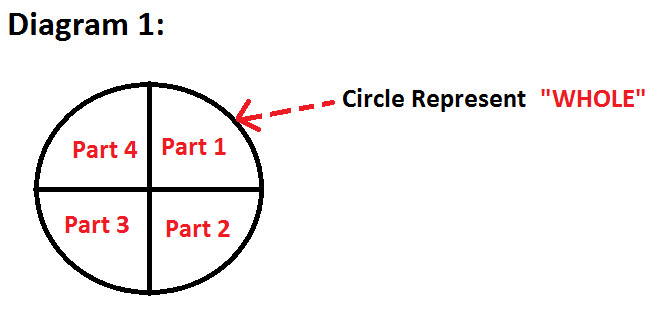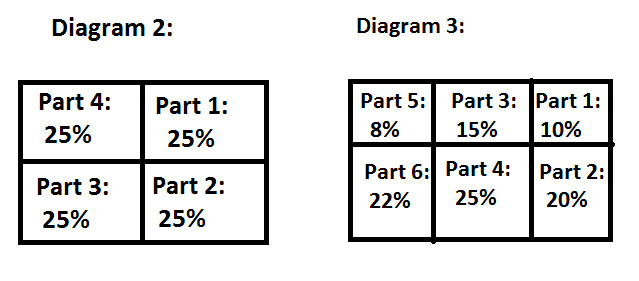Home >> Percentages >>

Define Percentage

Percentage to Fraction Percentage to Decimal Percentage Increase Percentage Decrease

Definition
The term Percentage was derived from two Latin Words Per and Centum which means Out of Hundred.
Percentage
is denoted by a symbol ' % '.

Percentage compares between the whole and its parts.
Before you understand, how Percentage compare between the whole and its parts; lets understand what is whole and what are its parts ?

Observe the following diagram; illustrating Whole and Its PartsAs you can observe in diagram 1:

• Whole is represented in the form of Circle.
• Part 1, Part 2, Part 3, Part 4 represents different parts of the whole

Or we can also write it as:
Whole Circle = Part 1 + Part 2 + Part 3 + Part 4

This means that sum of all the parts is equal to the whole or we can say that value of whole part is equal to the sum of values of all its part

Now let's understand this whole and its parts from a real life example:

Example: Four friends ordered a pizza. Pizza was divided into four equal portions. And each friend ate one portion.
Now in this situation:
Pizza represents the whole and four portions represent its 4 equal parts.

Now let's understand the significance of Percentage, which helps to find the value of the whole and its parts:

Value of whole part is always 100%
And as explained above, whole part is equal to sum of all parts; so:
This 100% value of whole part in divided into its different parts.

Observe the following diagram 2 & 3:Following are the observation from diagram 2 & 3:

• Value of whole in both the diagram is same i.e. 100%
• Sum of the values of parts in both the diagram is equal to 100% which is the value of whole
• Number of parts of a whole is not definite and it can vary from situation to situation
• Values of parts of the whole can be same as well as different also.

Now let's understand this whole and its parts from a real life example:

Example: In a class, there are 60% boys and 40% girls
Now, in this situation:
Class is a whole and boys & girls are its parts.
Also, values of parts i.e. boys and girls are 60% and 40% respectively.

Percentages can be :

• Converted to Fractions
• Converted to decimals
Explanation of these two topics(with examples) is available in the above links.

Study More Solved Questions / Examples